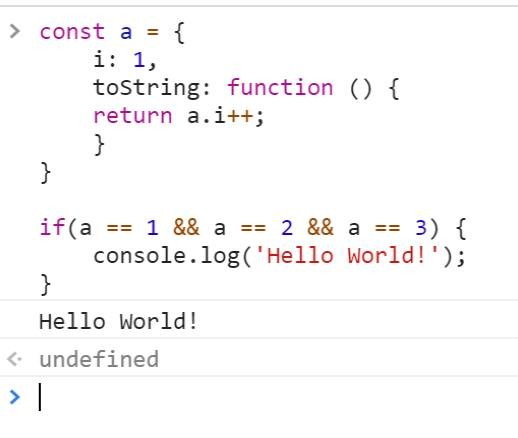#2018 iT 邦幫忙鐵人賽7
Modern Web

## 重新認識 JavaScript 番外篇 (7) - 判斷式 (a == 1 && a == 2 && a == 3) 結果為 true ？

Can (a ==1 && a== 2 && a==3) ever evaluate to true?

``````(a == 1 && a == 2 && a == 3)
``````.
.
.
.
.
.

``````const a = {
i: 1,
toString: function () {
return a.i++;
}
}

if(a == 1 && a == 2 && a == 3) {
console.log('Hello World!');
}
``````

``````if(a == 1 && a == 2 && a == 3) {
console.log('Hello World!');
}
``````

``````// 當 a == 1, a == 2, a == 3 都成立時才執行 console.log

if( a == 1 ) {
if( a == 2 ) {
if( a == 3 ) {
console.log('Hello World!');
}
}
}
``````

``````const a = {
i: 1,
toString: function () {
return a.i++;
}
}
``````

`a.i` 的初始值是 `1`，所以 `a.i++` 回傳的結果是什麼？ 還是 `1`。 (注意 `++` 的位置)

``````(a == 1 && a == 2 && a == 3) === true
``````### 1 則留言

0

``````(() => {
"use strict";
Object.defineProperty(this, "a", {
"get": () => {
Object.defineProperty(this, "a", {
"get": () => {
Object.defineProperty(this, "a", {
"get": () => {
return 3;
}
});
return 2;
},
configurable: true
});
return 1;
},
configurable: true
});
if (a == 1 && a == 2 && a == 3) {
document.body.append("Yes, it’s possible.");
}
})();
``````

Kuro Hsu iT邦新手 4 級 ‧ 2018-01-22 11:27:38 檢舉